# 1.本周学习总结

## 1.1思维导图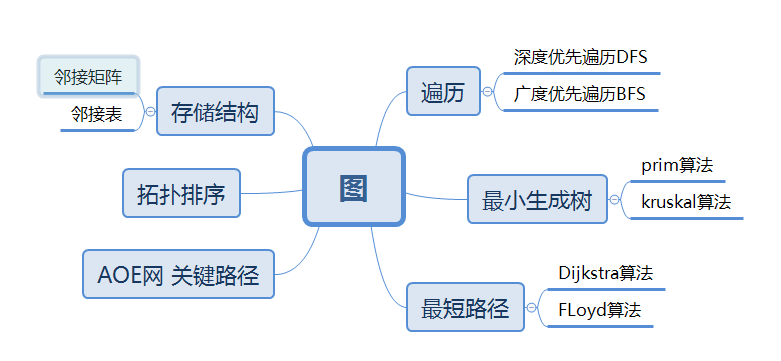# 2.PTA实验作业

## 2.1 图着色问题

### 2.1.1 设计思路

for i=0 to e
输入邻接表新节点
for i=0 to n
定义set类col存储颜色解种类
for j=1 to v
输入颜色存于数组color中
将该颜色存入col中
if check(v)==true 且 col.size()==k //利用set无视相同元素的特点
输出yes
else
输出no

bool check(int v)
for i=1 to v
for j=0 to G[i].size()
遍历邻接表比较与相邻颜色是否相同
return false //颜色相同
return true


### 2.1.2 代码截图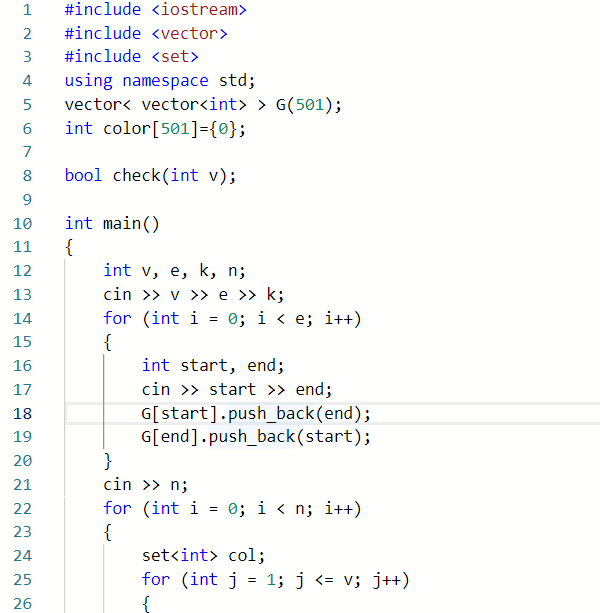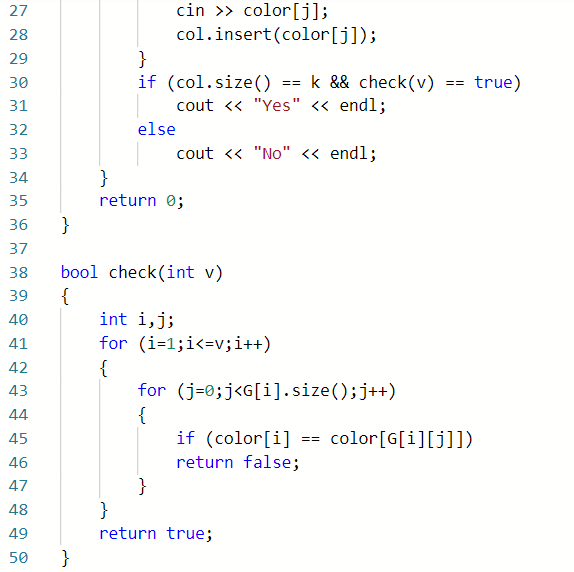### 2.1.3 本题PTA提交列表说明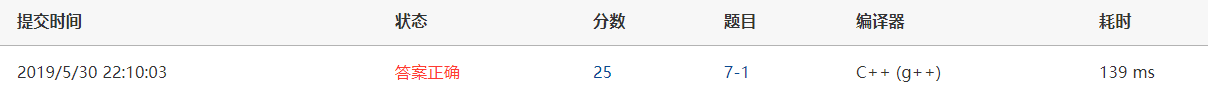## 2.2 旅游规划

### 2.2.1 设计思路




## 2.3 公路村村通

### 2.3.1 设计思路

输入n,e
if e>=n-1
for k=0 to e
输入顶点 权值
权值存入邻接矩阵
调用prim函数
else //一定不连通
输出-1

定义数组lowcost
for i=1 to n
lowcost赋初值
for i=0 to n
min=INF
for j=1 to n
if lowcost[j] 且 lowcost[j]<=min
min=lowcost[j];
k=j;
lowcost[k]=0
if min!=INF
count+1
cost+=min
for j=1 to n
修正lowcost数组
if count==n-1
输出cost
else //不连通
输出-1


### 2.3.2 代码截图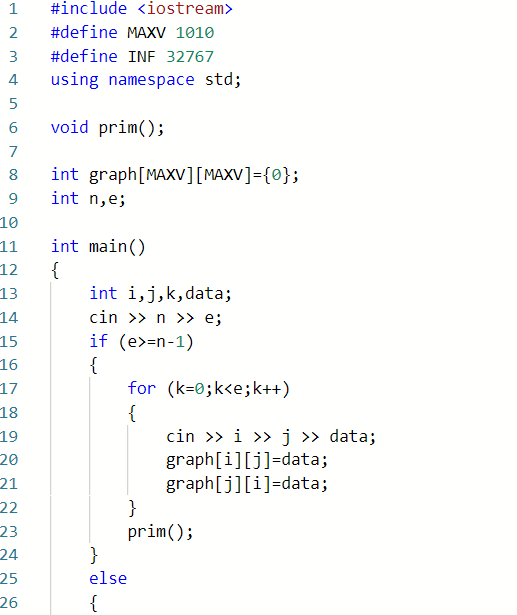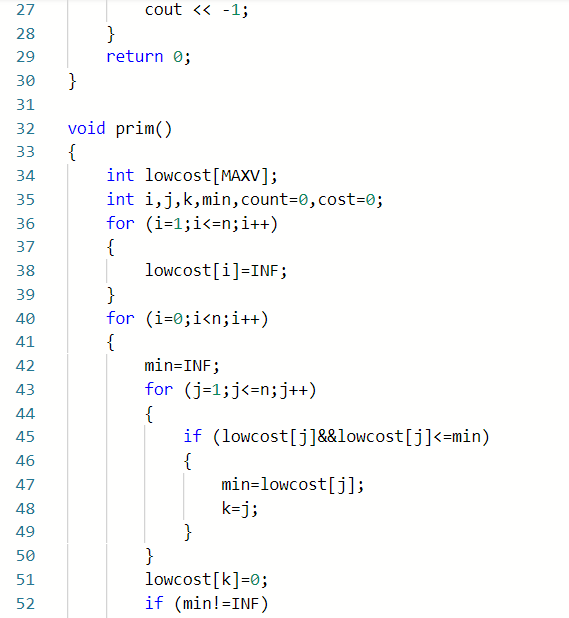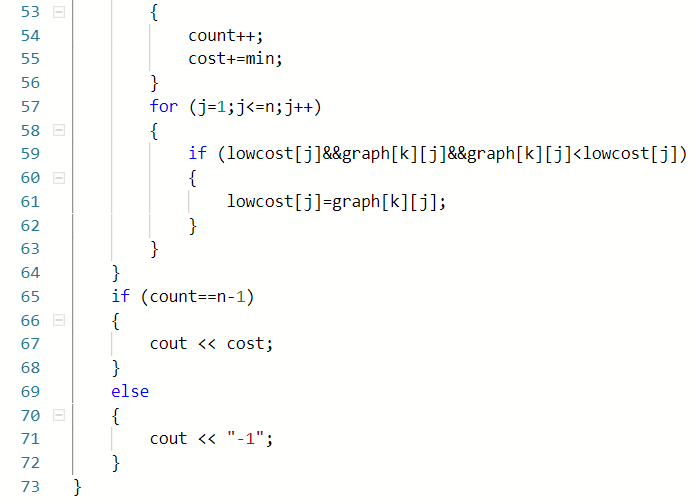### 2.3.3 本题PTA提交列表说明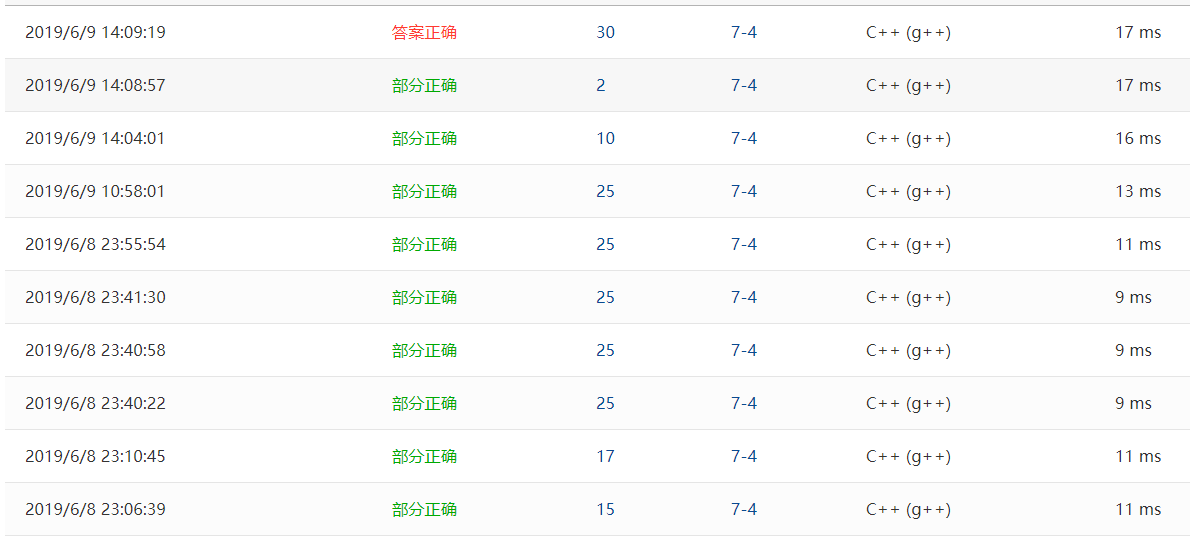Q1:count判定错误，所有非连通无法显示-1
A1:修正为count==n-1
Q2:M<N-1时段错误
A2:主函数中加入对该情况的判定
Q3:最大M，N答案错误
A3:找了好久直到写算法分析时发现lowcost数组初始化的问题# 3.上机考试错题及处理办法

## 3.1 六度空间

### 3.1.1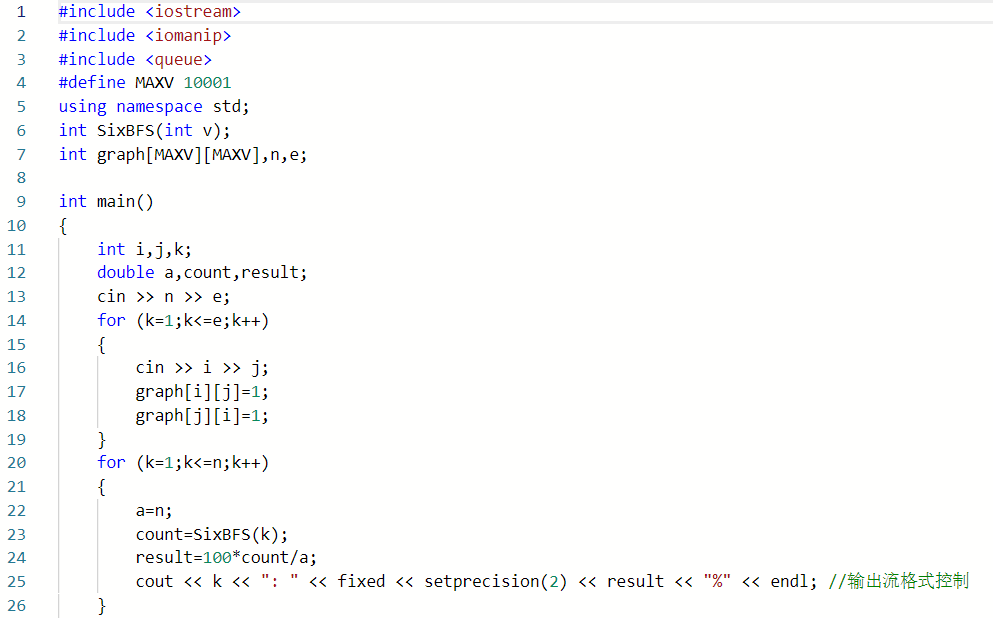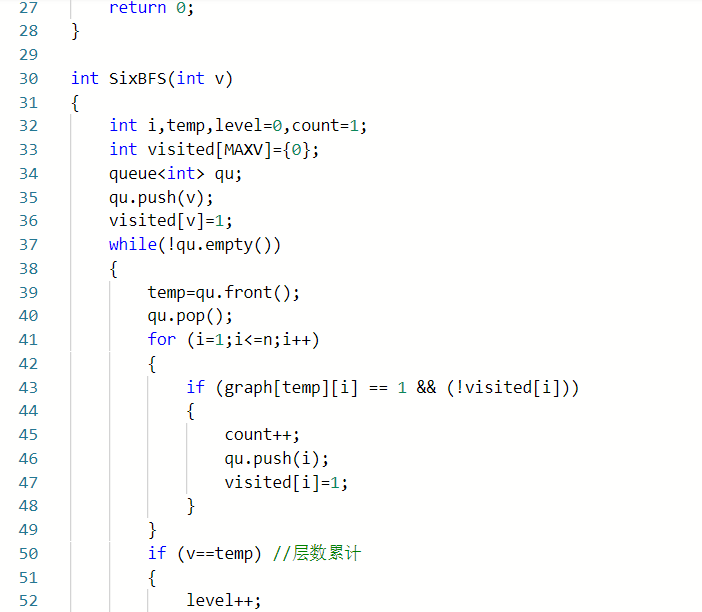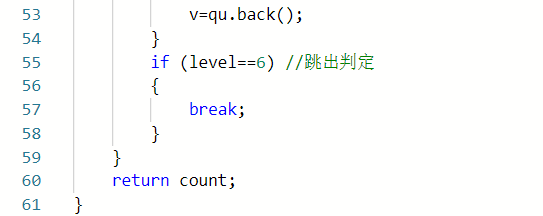## 3.2 公路村村通

### 3.2.2

posted @ 2019-06-02 20:24  ＫＩＳＡＭＫＯ  阅读(330)  评论(0编辑  收藏  举报